# verde.Chain¶

class verde.Chain(steps)[source]

Chain filtering operations to fit on each subsequent output.

The filter method of each element of the set is called with the outputs of the previous one. For gridders and trend estimators this means that each element fits the residuals (input data minus predicted data) of the previous one.

When predicting data, the predictions of each step in the chain are added together. Steps that don’t implement a predict method are ignored.

This provides a convenient way to chaining operations like trend estimation to a given gridder.

Parameters: steps : list A list of ('name', step) pairs where step is any verde class that implements a filter(coordinates, data, weights) method (including Chain itself).

verde.Vector
Fit an estimator to each component of multi-component vector data
Attributes: region_ : tuple The boundaries ([W, E, S, N]) of the data used to fit the chain. Used as the default region for the grid and scatter methods. named_steps : dict A dictionary version of steps.

Methods

 filter(coordinates, data[, weights]) Filter the data through the gridder and produce residuals. fit(coordinates, data[, weights]) Fit the chained estimators to the given data. get_params([deep]) Get parameters for this estimator. grid([region, shape, spacing, dims, …]) Interpolate the data onto a regular grid. predict(coordinates) Evaluates the data predicted by the chain on the given set of points. profile(point1, point2, size[, dims, …]) Interpolate data along a profile between two points. scatter([region, size, random_state, dims, …]) Interpolate values onto a random scatter of points. score(coordinates, data[, weights]) Score the gridder predictions against the given data. set_params(**params) Set the parameters of this estimator.

## Examples using verde.Chain¶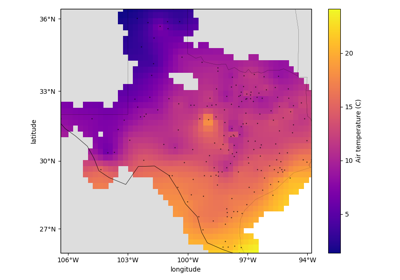Gridding with splines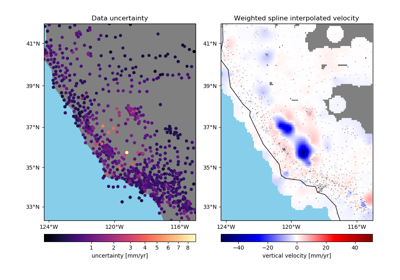Gridding with splines and weights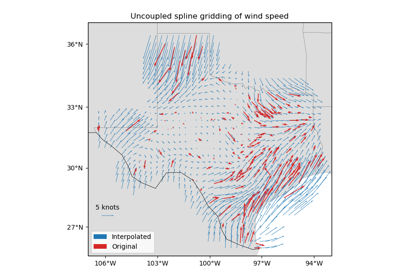Gridding 2D vectors (uncoupled)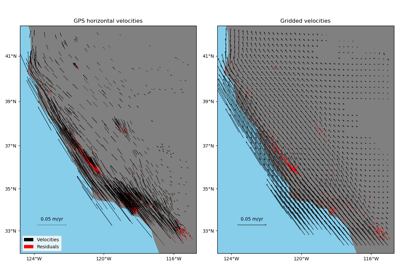Gridding 2D vectors (coupled)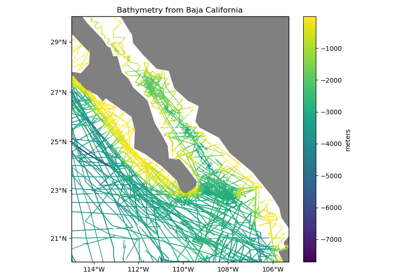Chaining Operations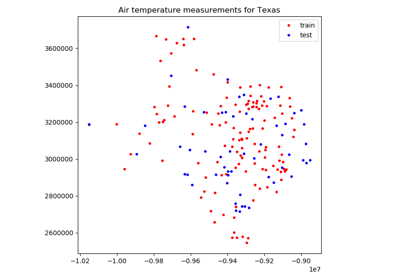Model Selection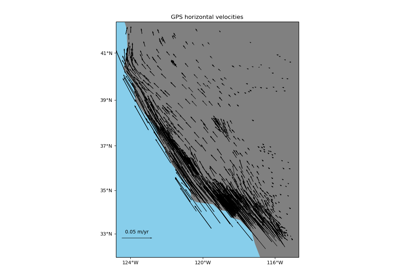Vector Data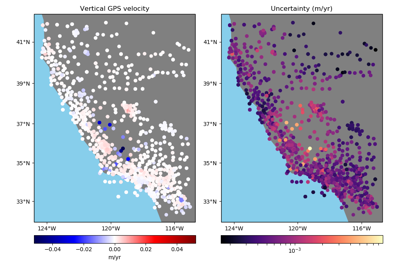Using Weights

Chain.filter(coordinates, data, weights=None)

Filter the data through the gridder and produce residuals.

Calls fit on the data, evaluates the residuals (data - predicted data), and returns the coordinates, residuals, and weights.

No very useful by itself but this interface makes gridders compatible with other processing operations and is used by verde.Chain to join them together (for example, so you can fit a spline on the residuals of a trend).

Parameters: coordinates : tuple of arrays Arrays with the coordinates of each data point. Should be in the following order: (easting, northing, vertical, …). data : array or tuple of arrays The data values of each data point. If the data has more than one component, data must be a tuple of arrays (one for each component). weights : None or array or tuple of arrays If not None, then the weights assigned to each data point. If more than one data component is provided, you must provide a weights array for each data component (if not None). coordinates, residuals, weights The coordinates and weights are same as the input. Residuals are the input data minus the predicted data.
Chain.fit(coordinates, data, weights=None)[source]

Fit the chained estimators to the given data.

Each estimator in the chain is fitted to the residuals of the previous estimator. The coordinates are preserved. Only the data values are changed.

The data region is captured and used as default for the grid and scatter methods.

All input arrays must have the same shape.

Parameters: coordinates : tuple of arrays Arrays with the coordinates of each data point. Should be in the following order: (easting, northing, vertical, …). data : array or tuple of arrays the data values of each data point. if the data has more than one component, data must be a tuple of arrays (one for each component). weights : none or array or tuple of arrays if not none, then the weights assigned to each data point. if more than one data component is provided, you must provide a weights array for each data component (if not none). self Returns this estimator instance for chaining operations.
Chain.get_params(deep=True)

Get parameters for this estimator.

Parameters: deep : boolean, optional If True, will return the parameters for this estimator and contained subobjects that are estimators. params : mapping of string to any Parameter names mapped to their values.
Chain.grid(region=None, shape=None, spacing=None, dims=None, data_names=None, projection=None, **kwargs)

Interpolate the data onto a regular grid.

The grid can be specified by either the number of points in each dimension (the shape) or by the grid node spacing. See verde.grid_coordinates for details. Other arguments for verde.grid_coordinates can be passed as extra keyword arguments (kwargs) to this method.

If the interpolator collected the input data region, then it will be used if region=None. Otherwise, you must specify the grid region.

Use the dims and data_names arguments to set custom names for the dimensions and the data field(s) in the output xarray.Dataset. Default names will be provided if none are given.

Parameters: region : list = [W, E, S, N] The boundaries of a given region in Cartesian or geographic coordinates. shape : tuple = (n_north, n_east) or None The number of points in the South-North and West-East directions, respectively. spacing : tuple = (s_north, s_east) or None The grid spacing in the South-North and West-East directions, respectively. dims : list or None The names of the northing and easting data dimensions, respectively, in the output grid. Defaults to ['northing', 'easting']. NOTE: This is an exception to the “easting” then “northing” pattern but is required for compatibility with xarray. data_names : list of None The name(s) of the data variables in the output grid. Defaults to ['scalars'] for scalar data, ['east_component', 'north_component'] for 2D vector data, and ['east_component', 'north_component', 'vertical_component'] for 3D vector data. projection : callable or None If not None, then should be a callable object projection(easting, northing) -> (proj_easting, proj_northing) that takes in easting and northing coordinate arrays and returns projected northing and easting coordinate arrays. This function will be used to project the generated grid coordinates before passing them into predict. For example, you can use this to generate a geographic grid from a Cartesian gridder. grid : xarray.Dataset The interpolated grid. Metadata about the interpolator is written to the attrs attribute.

verde.grid_coordinates
Generate the coordinate values for the grid.
Chain.predict(coordinates)[source]

Evaluates the data predicted by the chain on the given set of points.

Predictions from each step in the chain are added together. Any step that doesn’t implement a predict method is ignored.

Requires a fitted gridder (see fit).

Parameters: coordinates : tuple of arrays Arrays with the coordinates of each data point. Should be in the following order: (easting, northing, vertical, …). data : array The data values predicted on the given points.
Chain.profile(point1, point2, size, dims=None, data_names=None, projection=None, **kwargs)

Interpolate data along a profile between two points.

Generates the profile along a straight line assuming Cartesian distances. Point coordinates are generated by verde.profile_coordinates. Other arguments for this function can be passed as extra keyword arguments (kwargs) to this method.

Use the dims and data_names arguments to set custom names for the dimensions and the data field(s) in the output pandas.DataFrame. Default names are provided.

Includes the calculated Cartesian distance from point1 for each data point in the profile.

Parameters: point1 : tuple The easting and northing coordinates, respectively, of the first point. point2 : tuple The easting and northing coordinates, respectively, of the second point. size : int The number of points to generate. dims : list or None The names of the northing and easting data dimensions, respectively, in the output dataframe. Defaults to ['northing', 'easting']. NOTE: This is an exception to the “easting” then “northing” pattern but is required for compatibility with xarray. data_names : list of None The name(s) of the data variables in the output dataframe. Defaults to ['scalars'] for scalar data, ['east_component', 'north_component'] for 2D vector data, and ['east_component', 'north_component', 'vertical_component'] for 3D vector data. projection : callable or None If not None, then should be a callable object projection(easting, northing) -> (proj_easting, proj_northing) that takes in easting and northing coordinate arrays and returns projected northing and easting coordinate arrays. This function will be used to project the generated profile coordinates before passing them into predict. For example, you can use this to generate a geographic profile from a Cartesian gridder. table : pandas.DataFrame The interpolated values along the profile.
Chain.scatter(region=None, size=300, random_state=0, dims=None, data_names=None, projection=None, **kwargs)

Interpolate values onto a random scatter of points.

Point coordinates are generated by verde.scatter_points. Other arguments for this function can be passed as extra keyword arguments (kwargs) to this method.

If the interpolator collected the input data region, then it will be used if region=None. Otherwise, you must specify the grid region.

Use the dims and data_names arguments to set custom names for the dimensions and the data field(s) in the output pandas.DataFrame. Default names are provided.

Parameters: region : list = [W, E, S, N] The boundaries of a given region in Cartesian or geographic coordinates. size : int The number of points to generate. random_state : numpy.random.RandomState or an int seed A random number generator used to define the state of the random permutations. Use a fixed seed to make sure computations are reproducible. Use None to choose a seed automatically (resulting in different numbers with each run). dims : list or None The names of the northing and easting data dimensions, respectively, in the output dataframe. Defaults to ['northing', 'easting']. NOTE: This is an exception to the “easting” then “northing” pattern but is required for compatibility with xarray. data_names : list of None The name(s) of the data variables in the output dataframe. Defaults to ['scalars'] for scalar data, ['east_component', 'north_component'] for 2D vector data, and ['east_component', 'north_component', 'vertical_component'] for 3D vector data. projection : callable or None If not None, then should be a callable object projection(easting, northing) -> (proj_easting, proj_northing) that takes in easting and northing coordinate arrays and returns projected northing and easting coordinate arrays. This function will be used to project the generated scatter coordinates before passing them into predict. For example, you can use this to generate a geographic scatter from a Cartesian gridder. table : pandas.DataFrame The interpolated values on a random set of points.
Chain.score(coordinates, data, weights=None)

Score the gridder predictions against the given data.

Calculates the R^2 coefficient of determination of between the predicted values and the given data values. A maximum score of 1 means a perfect fit. The score can be negative.

If the data has more than 1 component, the scores of each component will be averaged.

Parameters: coordinates : tuple of arrays Arrays with the coordinates of each data point. Should be in the following order: (easting, northing, vertical, …). data : array or tuple of arrays The data values of each data point. If the data has more than one component, data must be a tuple of arrays (one for each component). weights : None or array or tuple of arrays If not None, then the weights assigned to each data point. If more than one data component is provided, you must provide a weights array for each data component (if not None). score : float The R^2 score
Chain.set_params(**params)

Set the parameters of this estimator.

The method works on simple estimators as well as on nested objects (such as pipelines). The latter have parameters of the form <component>__<parameter> so that it’s possible to update each component of a nested object.

Returns: self